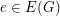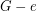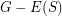Arthur

3-Edge-Coloring Conjecture ★★★

Author(s): Arthur; Hoffmann-Ostenhof

Conjecture   Supposewithis a connected cubic graph admitting a-edge coloring. Then there is an edgesuch that the cubic graph homeomorphic tohas a-edge coloring.

Keywords: 3-edge coloring; 4-flow; removable edge

Cycle Double Covers Containing Predefined 2-Regular Subgraphs ★★★

Author(s): Arthur; Hoffmann-Ostenhof

Conjecture   Letbe a-connected cubic graph and letbe a-regular subgraph such thatis connected. Thenhas a cycle double cover which contains(i.e all cycles of).

Keywords:

3-Decomposition Conjecture ★★★

Author(s): Arthur; Hoffmann-Ostenhof

Conjecture   (3-Decomposition Conjecture) Every connected cubic graphhas a decomposition into a spanning tree, a family of cycles and a matching.

Keywords: cubic graph

Strong 5-cycle double cover conjecture ★★★

Author(s): Arthur; Hoffmann-Ostenhof

Conjecture   Letbe a circuit in a bridgeless cubic graph. Then there is a five cycle double cover ofsuch thatis a subgraph of one of these five cycles.

Keywords: cycle cover